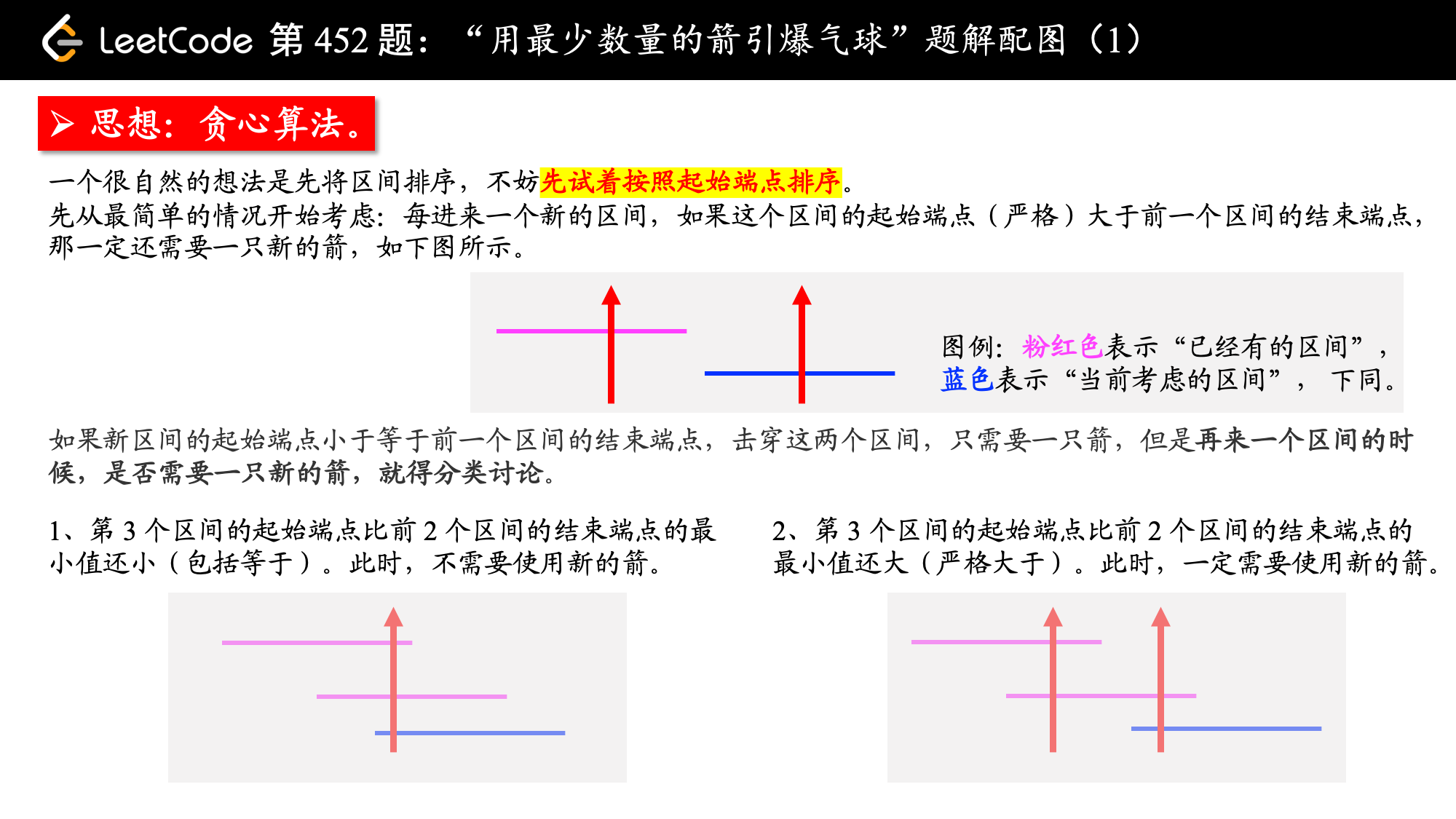# 「力扣」第 452 题：用最少数量的箭引爆气球

## 「力扣」第 452 题：用最少数量的箭引爆气球

Example:

[[10,16], [2,8], [1,6], [7,12]]

2

## 贪心算法（Python 代码、Java 代码）Python 代码：

class Solution:
def findMinArrowShots(self, points: List[List[int]]) -> int:
size = len(points)
# 特判
if size < 2:
return size
# 按照区间的起始端点排序
points.sort(key=lambda x:x)

# 只要有区间就至少需要一只箭
res = 1
# 最远距离：使用当前这只箭能引爆气球的最远距离
end = points

for i in range(1, size):
if points[i] > end:
end = points[i]
res += 1
else:
end = min(end, points[i])
return res

Java 代码：

import java.util.Arrays;
import java.util.Comparator;

public class Solution {

public int findMinArrowShots(int[][] points) {
int plen = points.length;
if (plen < 2) {
return plen;
}

// 按照起点进行排序
Arrays.sort(points, new Comparator() {
@Override
public int compare(int[] o1, int[] o2) {
if (o1 != o2) {
return o1 - o2;
}
return o1 - o2;
}
});

// 最少的数量
int minCount = 1;
// 第 1 个区间的末尾，目前线段能够达到的最远位置
int end = points;
// 贪心法，基于上一个箭，记录当前能够射穿的所有的区间
for (int i = 1; i < plen; i++) {
if (points[i] <= end) {
end = Math.min(end, points[i]);
} else {
minCount++;
end = points[i];
}
}
return minCount;
}
}

（如果上面的叙述不好理解的话，可以在草稿纸上多画几条线段，就很清楚了。）

Python 代码：

class Solution:
def findMinArrowShots(self, points: List[List[int]]) -> int:
size = len(points)
if size < 2:
return size

# 按照区间的末尾端点排序
points.sort(key=lambda x:x)
res = 1
# 最远距离：使用当前这只箭能引爆气球的最远距离
end = points

for i in range(1, size):
if points[i] > end:
end = points[i]
res += 1
return res

Java 代码：

import java.util.Arrays;
import java.util.Comparator;

public class Solution {
public int findMinArrowShots(int[][] points) {
int len = points.length;
if (len < 2) {
return len;
}

// 按照区间终点进行排序
Arrays.sort(points, new Comparator() {
@Override
public int compare(int[] point1, int[] point2) {
if (point1 != point2) {
return point1 - point2;
}
return point1 - point2;
}
});

int count = 1;
int end = points;
for (int i = 1; i < len; i++) {
if (points[i] > end) {
// 就得多用一支箭
end = points[i];
count++;
}
}
return count;
}
}

• 时间复杂度：$O(N \log N)$：$N$ 为气球的个数，时间复杂度为排序算法的时间复杂度，感谢用户 @powerboy6 提供的评论。
• 空间复杂度：$O(1)$。

上一篇「力扣」第 455 题：分发饼干
「力扣」第 455 题：分发饼干题解地址：贪心算法（两个方向） + 优先队列（Python 代码）。 说明：文本首发在力扣的题解版块，更新也会在第 1 时间在上面的网站中更新，这篇文章只是上面的文章的一个快照，您可以点击上面的链接看到其他网
2018-07-07「力扣」第 354 题：俄罗斯套娃信封问题
「力扣」第 354 题：俄罗斯套娃信封问题题解地址：贪心算法、二分查找（Python 代码、Java 代码）。 说明：文本首发在力扣的题解版块，更新也会在第 1 时间在上面的网站中更新，这篇文章只是上面的文章的一个快照，您可以点击上面的链接
2018-07-05
目录